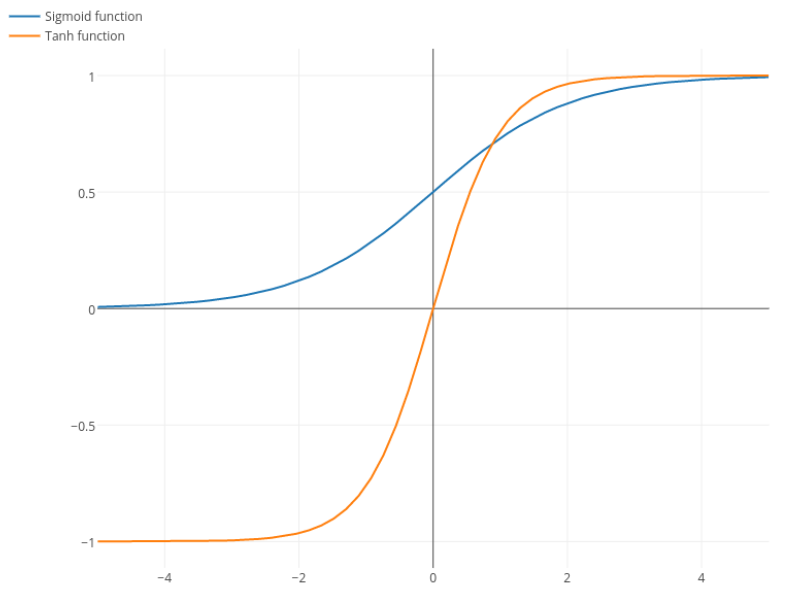# Sigmoid Function

## What Is A Sigmoid Function?

A sigmoid function is a type of activation function, and more specifically defined as a squashing function. Squashing functions limit the output to a range between 0 and 1, making these functions useful in the prediction of probabilities.

The name Sigmoidal comes from the Greek letter Sigma, and when graphed, appears as a sloping “S” across the Y-axis. A sigmoidal function is a type of logistic function and purely refers to any function that retains the “S” shape, such as tanh(x). Where a traditional sigmoidal function exists between 0 and 1, tanh(x) follows a similar shape, but exists between 1 and -1. On its own, a sigmoidal function is also differentiable, meaning we can find the slope of the sigmoid curve, at any two points.Image from stackexchange.com

## Sigmoid Functions in Machine Learning

Sigmoidal functions are frequently used in machine learning, specifically in the testing of artificial neural networks, as a way of understanding the output of a node or “neuron.” For example, a neural network may attempt to find a desired solution given a set of inputs. A sigmoidal function will determine the output and that output will be used as the input for the following node. This process will repeat until the solution to the original problem is found.